# Texas Go Math Grade 4 Lesson 2.6 Answer Key Compare Decimals

Refer to our Texas Go Math Grade 4 Answer Key Pdf to score good marks in the exams. Test yourself by practicing the problems from Texas Go Math Grade 4 Lesson 2.6 Answer Key Compare Decimals.

## Texas Go Math Grade 4 Lesson 2.6 Answer Key Compare Decimals

Essential Question

How can you compare decimals?
The rules for comparing decimal numbers are as follows:
a. Compare the whole number part first (i.e., compare the digits in front of the decimal). If they are different, then compare the numbers as you would for any whole number.
b. Compare the tenth place.
c. Compare the hundredth place.
Example:Unlock the Problem:

The city park covers 0.64 square miles. About 0.18 of the park is covered by water, and about 0.2 of the park is covered by paved walkways. Is more of the park covered by water or paved walkways?

• Cross out unnecessary information.
• Circle numbers you will use.
• What do you need to find?

One Way Use a model.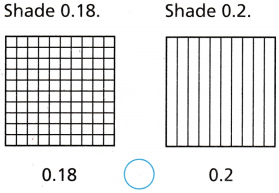We know that,
The equivalent of 0.2 is: 0.20
Now,
Compare 0.18 and 0.20
So,
From the rules of comparing decimals,
18 < 20
Hence, from the above,
We can conclude that
The representation of the model with the comparison of the given decimal numbers is: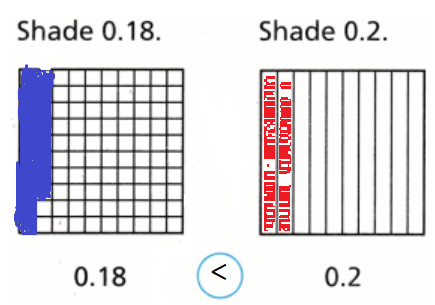Other Ways

A. Use a number line.

Locate 0.18 and 0.2 on a number line.
Think: 2 tenths is equivalent to 20 hundredths.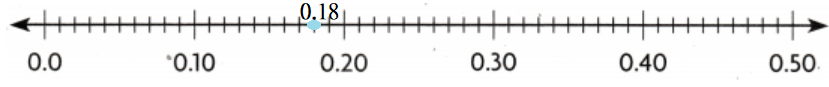Now,
From the above Number line,
We can observe that
0.18 is closer to 0
So,
0.18 < 0.2.

B. Compare equal-size parts.

• 0.18 is hundredths.
• 0.2 is 2 tenths, which is equivalent to 20 hundredths.

We know that,
18 hundredths < 20 hundredths
So 0.18 < 0.2.
Hence, from the above,
We can conclude that
More of the park is covered by “Paved Walkways”

Math Talk

Mathematical Processes
How does the number of tenths in 0.18 compare to the number of tenths in 0.2? Explain.
The given decimal numbers are: 0.18 and 0.2
Now,
The representation of 0.18 in the place-value chart is:The representation of 0.2 in the place-value chart is:Now,
From the above place-value charts,
The comparison of the tenth place of 0.18 and 0.2 is:
1 < 2
Hence, from the above,
We can conclude that
The number of tenths in 0.18 < The number of tenths in 0.2

Example Use Place value.

Tim has 0.5 dollars and Sienna has 0.05 dollars. Who has more money?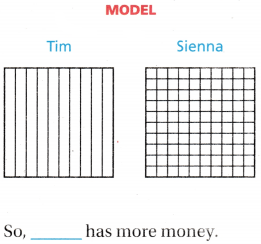It is given that
Tim has 0.5 dollars and Sienna has 0.05 dollars.
Hence, from the above,
We can conclude that
The representation of the models of the given decimal numbers is:It is given that
Tim has 0.5 dollars and Sienna has 0.05 dollars.
Hence, from the above,
We can conclude that
The representation of the record of the given decimal numbers is: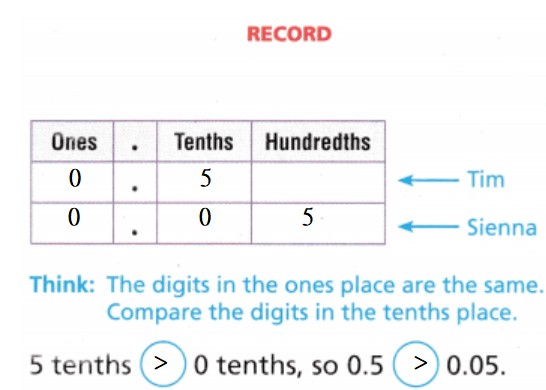Share and Show

Question 1.
Compare 0.39 and 0.42. Write <, >, or = Shade the model to help.0.39 __________ 0.42
The given decimal numbers are: 0.39 and 0.42
Now,
The representation of the models represented by 0.39 and 0.42 is: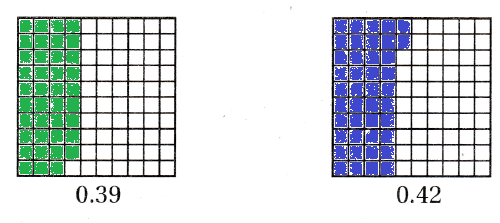So,
From the above models,
We can observe that
0.39 < 0.42
Hence, from the above,
We can conclude thatCompare. Write <, >, or =.

Question 2.
4.5 ____________ 2.89The given decimal numbers are: 4.5 and 2.89
Now,
The representation of the given decimal numbers in the place-value chart is: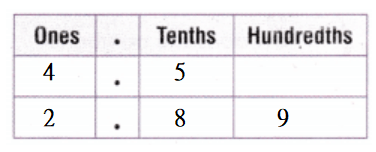Now,
From the above place-value charts,
We can observe that
According to the rules of comparing decimals,
4 > 2
Hence, from the above,
We can conclude that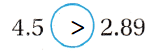Question 3.
0.7 _____________ 0.54The given decimal numbers are: 0.7 and 0.54
Now,
The representation of the given decimal numbers in the place-value chart is:Now,
From the above place-value charts,
We can observe that
According to the rules of comparing decimals,
0.7 > 0.2
Hence, from the above,
We can conclude that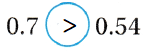Math Talk

Mathematical Processes

Can you compare 0.39 and 0.42 by comparing only the tenths? Explain.
The given decimal numbers are: 0.39 and 0.42
Now,
We know that,
In the comparison of decimals,
If the whole numbers before the decimal point are the same, then compare the tenths of the decimal numbers
Now,
The representation of 0.39 and 0.42 in the place-value chart is:Now,
From the above place-value charts,
We can observe that
3 tenths < 4 tenths
Hence, from the above,
We can conclude that
3 tenths in 0.39 < 4 tenths in 0.42

Unlock the problem

Question 4.
H.O.T. Multi-Step Ricardo and Brandon ran a 1500-meter race. Ricardo finished in 4.89 minutes. Brandon finished in 4.83 minutes. What was the time of the runner who finished first?
(A) 15.00 minutes
(B) 4.83 minutes
(C) 4.89 minutes
(D) Ricardo and Brandon tied for first.

a. What are you asked to find?
It is given that
Ricardo and Brandon ran a 1500-meter race. Ricardo finished in 4.89 minutes. Brandon finished in 4.83 minutes
Hence, from the above,
We can conclude that
You are asked to find the time of the runner who finished first

b. What do you need to do to find the answer?
We need to compare the place values of the given decimal numbers. If a place-value is equal then compare the next place-vale and so on

C. Solve the problem.
The representation of 4.89 and 4.83 in the place-value chart is:Now,
From the above place-value chart,
We can observe that
9 hundredths in 4.89 > 3 hundredths in 4.83
Hence, from the above,
We can conclude that
The time of the runner who finished first is: 4.89 minutes

d. Fill in the bubble for the correct answer choice above.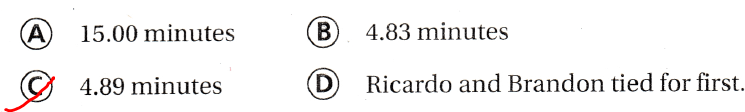Yes, the answer makes sense because we followed the rules of the comparison of decimal numbers

Question 5.
Multi-Step Jack had $4.53 in his pocket. Sam had$14.25 in his pocket. Jill had $14.40 in her pocket. Who had more money, Jack or Jill? Did Sam have more money than either Jack or Jill? Answer: It is given that Jack had$4.53 in his pocket. Sam had $14.25 in his pocket. Jill had$14.40 in her pocket.
Now,
The representation of $4.53 in the place-value chart is: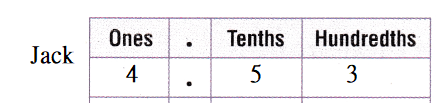The representation of$14.25 in the place-value chart is: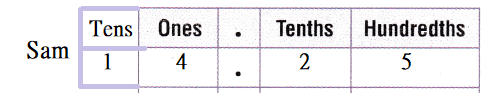The representation of $14.40 in the place-value chart is: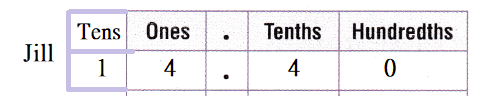Now, From the above place-value charts, We can observe that Jack has more money Sam did not have more money than either Jack or Jill Hence, from the above, We can conclude that Jack has more money Sam did not have more money than either Jack or Jill Daily Assessment Task Fill in the bubble completely to show your answer. Question 6. Multi-Step The Venus flytrap closes in 0.3 seconds and the waterwheel plant closes in 0.2 seconds. Which decimal is less than 0.3 but greater than 0.2? (A) 0.01 (B) 0.32 (C) 0.21 (D) 0.4 Answer: It is given that The Venus flytrap closes in 0.3 seconds and the waterwheel plant closes in 0.2 seconds Now, The given decimal numbers are: 0.3 and 0.2 Now, The decimal numbers that are greater than 0.2 but less than 0.3 are: 0.21, 0.22, 0.23, 0.24, 0.25, 0.26, 0.27, 0.28, 0.29 Hence, from the above, We can conclude that The decimal that is less than 0.3 but greater than 0.2 is:Question 7. Representations Which model represents a number greater than 0.65? (A)(B)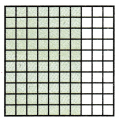(C)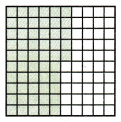(D)Answer: The given decimal number is: 0.65 So, The representation of 0.65 in the fraction form is: $$\frac{65}{100}$$ So, In the model, The total number of parts are: 100 The number of shaded parts is: 65 So, To represent a model that represents a number greater than 0.65, The number of shaded parts will be greater than 65 Hence, from the above, We can conclude that The model that represents a model that is greater than 0.65 is:Question 8. Use Symbols Compare 0.24 and 0.3. Which statement is true?(A) 0.24 > 0.3 (B) 0.24 0.3 (C) 0.3 <0.24 (D) 0.24 < 0.3 Answer: The given decimal numbers are: 0.24 and 0.3 Now, The given number line is:Now, The representation of the given decimal numbers in the number line is: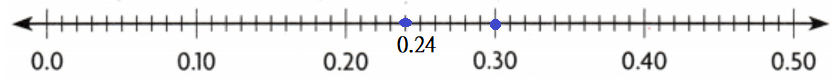Now, From the above number line, We can observe that 0.24 is close to 0 So, 0.24 < 0.30 Hence, from the above, We can conclude that The statement that is true is: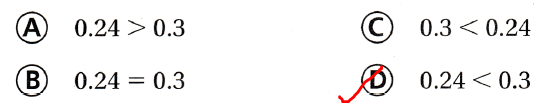TEXAS Test Prep Question 9. Maria has$3.68 in her pocket. Dennis has less money in his pocket than Maria does. Which could be an amount that Dennis has in his pocket?
(A) $3.60 (B)$3.78
(C) $7.38 (D)$13.24
It is given that
Maria has $3.68 in her pocket. Dennis has less money in his pocket than Maria does Now, According to the given information, The money that Dennis has should be less than$3.68
Hence, from the above,
We can conclude that
The amount that Dennis has in his pocket is:### Texas Go Math Grade 4 Lesson 2.6 Homework and Practice Answer Key

Compare Decimals

Compare. Write <, >, or =. For 1-2, complete the table.

Question 1.
0.26 _______ 0.23The given decimal numbers are: 0.26 and 0.23
Now,
The representation of the given decimal numbers in the place-value chart is: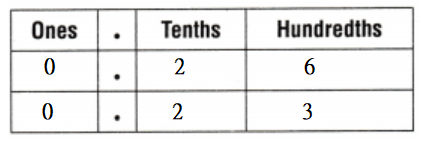Now,
From the above place-value charts,
We can observe that
According to the rules of comparing decimals,
6 > 3
Hence, from the above,
We can conclude that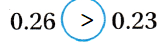Question 2.
1.15 _______ 1.3The given decimal numbers are: 1.15 and 1.3
Now,
The representation of the given decimal numbers in the place-value chart is:Now,
From the above place-value charts,
We can observe that
According to the rules of comparing decimals,
1 tenth < 3 tenth
Hence, from the above,
We can conclude thatQuestion 3.
0.9 _______ 0.81
The given decimal numbers are: 0.9 and 0.81
Now,
The representation of the given decimal numbers in the place-value chart is: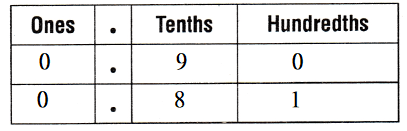Now,
From the above place-value charts,
We can observe that
According to the rules of comparing decimals,
9 tenths > 8 tenths
Hence, from the above,
We can conclude thatQuestion 4.
1.06 _______ 0.6
The given decimal numbers are: 1.06 and 0.6
Now,
The representation of the given decimal numbers in the place-value chart is: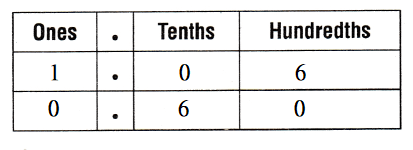Now,
From the above place-value charts,
We can observe that
According to the rules of comparing decimals,
1 > 0
Hence, from the above,
We can conclude thatQuestion 5.
0.25 _______ 0.3
The given decimal numbers are: 0.25 and 0.3
Now,
The representation of the given decimal numbers in the place-value chart is:Now,
From the above place-value charts,
We can observe that
According to the rules of comparing decimals,
2 tenths < 3 tenths
Hence, from the above,
We can conclude thatQuestion 6.
2.61 _______ 3.29
The given decimal numbers are: 2.61 and 3.29
Now,
The representation of the given decimal numbers in the place-value chart is: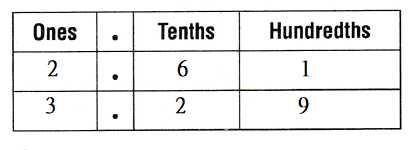Now,
From the above place-value charts,
We can observe that
According to the rules of comparing decimals,
2 < 3
Hence, from the above,
We can conclude that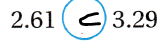Question 7.
0.38 _______ 0.83
The given decimal numbers are: 0.38 and 0.83
Now,
The representation of the given decimal numbers in the place-value chart is:Now,
From the above place-value charts,
We can observe that
According to the rules of comparing decimals,
3 tenths < 8 tenths
Hence, from the above,
We can conclude that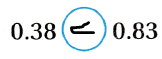Question 8.
1.9 _______ 0.99
The given decimal numbers are: 1.9 and 0.99
Now,
The representation of the given decimal numbers in the place-value chart is: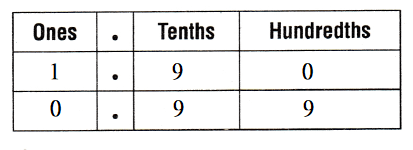Now,
From the above place-value charts,
We can observe that
According to the rules of comparing decimals,
1 > 0
Hence, from the above,
We can conclude that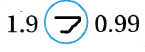Question 9.
1.11 _______ 1.41
The given decimal numbers are: 1.11 and 1.41
Now,
The representation of the given decimal numbers in the place-value chart is:Now,
From the above place-value charts,
We can observe that
According to the rules of comparing decimals,
1 tenth < 4 tenths
Hence, from the above,
We can conclude that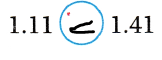Question 10.
0.8 _______ 0.80
The given decimal numbers are: 0.8 and 0.80
Now,
The representation of the given decimal numbers in the place-value chart is:Now,
From the above place-value charts,
We can observe that
According to the rules of comparing decimals,
8 tenths = 8 tenths
Hence, from the above,
We can conclude thatProblem Solving

Question 11.
Neil and Anne each bought a book at a used book store. Neil paid $5.37 for his book. Anne paid$5.32 for her book. Who spent more money?
It is given that
Neil and Anne each bought a book at a used book store. Neil paid $5.37 for his book. Anne paid$5.32 for her book.
Now,
The given decimal numbers are: 5.37 and 5.32
Now,
The representation of the given decimal numbers in the place-value chart is: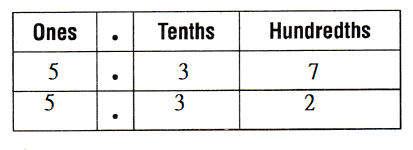Now,
From the above place-value chart,
7 hundredths > 2 hundredths
So,
5.37 > 5.32
Hence, from the above,
We can conclude that
Neil spent more money

Question 12.
Lynn drove 4.06 miles to the store. Patrick drove 4.6 miles to the library. Who drove farther?
It is given that
Lynn drove 4.06 miles to the store. Patrick drove 4.6 miles to the library
Now,
The given decimal numbers are: 4.06 and 4.6
Now,
The representation of the given decimal numbers in the place-value chart is: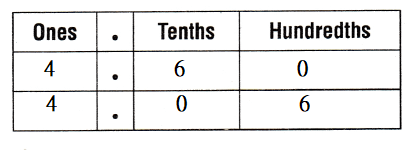Now,
From the above place-value chart,
6 tenths > 0 tenths
So,
4.60 > 4.06
Hence, from the above,
We can conclude that
Patrick drove farther

Question 13.
Barbara has $3.43 in her pocket. Kendra has$3.34 in her bank. Who has the greater amount of money?
It is given that
Barbara has $3.43 in her pocket. Kendra has$3.34 in her bank
Now,
The given decimal numbers are: 3.43 and 3.34
Now,
The representation of the given decimal numbers in the place-value chart is: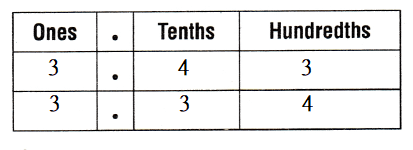Now,
From the above place-value chart,
4 tenths > 3 tenths
So,
3.43 > 3.34
Hence, from the above,
We can conclude that
Barbara has the greater amount of money

Question 14.
Jordan and Shelly were raising money by walking around a track. Jordan raised $47.50 and Shelly raised$45.50. Who raised more money?
It is given that
Jordan and Shelly were raising money by walking around a track. Jordan raised $47.50 and Shelly raised$45.50
Now,
The given decimal numbers are: 47.50 and 45.50
Now,
The representation of the given decimal numbers in the place-value chart is:Now,
From the above place-value chart,
7 > 5
So,
47.50 > 45.50
Hence, from the above,
We can conclude that
Jordan raised more money

Lesson Check

Question 15.
Which model represents a number less than 0.27?
(A)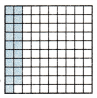(B)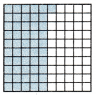(C)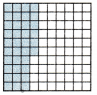(D)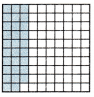The given decimal number is: 0.27
Now,
The representation of 0.27 in the form of a fraction is: $$\frac{27}{100}$$
So,
To represent the model that is less than 0.27,
The shaded parts must be 27
Hence, from the above,
We can conclude that
The model represents a number less than 0.27 is:Question 16.
Compare 0.3 and 0.28. Which statement is true?(A) 0.3 > 0.28
(B) 0.28 = 0.3
(C) 0.28 > 0.3
(D) 0.3 < 0.28
The given decimal numbers are: 0.3 and 0.28
Now,
The given number line is:Now,
The representation of the given decimal numbers in the number line is: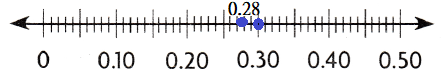Now,
From the above number line,
We can observe that
0.28 is closer to 0
So,
0.28 < 0.3
Hence, from the above,
We can conclude that
The statement that is true is: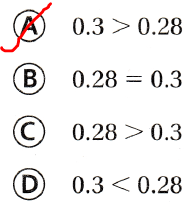Question 17.
Jake’s time for the 100-meter race was 11.46 seconds. Dan’s time was faster. Which could he Dan’s time?
(A) 11.55 seconds
(B) 11.73 seconds
(C) 11.38 seconds
(D) 11.49 seconds
It is given that
Jake’s time for the 100-meter race was 11.46 seconds. Dan’s time was faster
Now,
According to the given information,
Dan’s time must be lesser than 11.46 seconds
Hence, from the above,
We can conclude that
Dan’s time could be: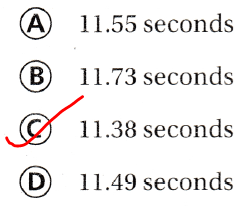Question 18.
Which of the following is less than 19.45?
(A) 19.54
(B) 19.44
(C) 20.64
(D) 20.53
The given decimal number is: 19.45
Hence, from the above,
We can conclude that
The number is less than 19.45 is:Question 19.
Multi-Step Which of the following is more than $4.72 and less than$4.93?
(A) $4.81 (B)$3.99
(C) $4.68 (D)$3.89
The given decimal numbers are: 4.72 and 4.93
Now,
According to the given information,
The numbers that are more than 4.72 and less than 4.93 are: 4.73 to 4.92
Hence, from the above,
We can conclude that
The number is more than $4.72 and less than$4.93 is: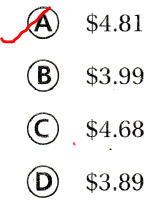Question 20.
Multi-Step Wayne has $6.83. Christie has$6.55. Kareem has $6.79. Which statement is true? (A) Kareem has the least money. (B) Christie has more money than Kareem. (C) Wayne has less money than Christie. (D) Wayne has the most money. Answer: It is given that Wayne has$6.83. Christie has $6.55. Kareem has$6.79
Now,
The given decimal numbers are: 6.83, 6.55, and 6.79
Now,
Compare the tenth place value of the given decimal numbers
8 tenths > 7 tenths > 5 tenths
Hence, from the above,
We can conclude that
The statement that is true is:Scroll to Top